﻿ 2D Shapes
 HOME ABOUT US SHOP PRIVACY CONTACT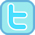# 2D Shapes

 Our printable resources...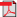Quadrilaterals Sheet 1          Sheet 2          Sheet 3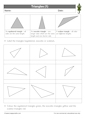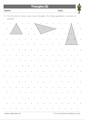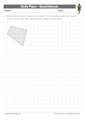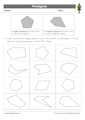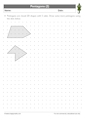Equilateral, Isosceles and Scalene TrianglesIsometric TrianglesIdentifying QuadrilateralsDotty paper - QuadrilateralsIdentifying PentagonsDotty Paper - Pentagons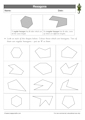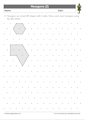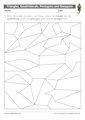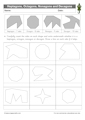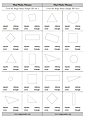Identifying HexagonsIsometric Paper - HexagonsTriangles, Quadrilaterals, Pentagons and HexagonsHeptagons, Octagons, Nonagons and DecagonsSimple 2D Shape Mad Maths Minutes Sets A & BSimple 2D Shape Mad Maths Minutes Sets C & D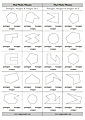Pentagon, Hexagon or Octagon Mad Maths Minutes Sets A & BPentagon, Hexagon or Octagon Mad Maths Minutes Sets C & DHow Many Sides Mad Maths Minutes Sets A & BHow Many Sides Mad Maths Minutes Sets C & DOur online activities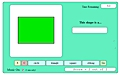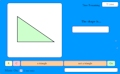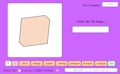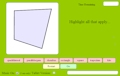Simple 2D Shape Interactive Mad Maths (circle, triangle, square, oblong)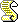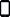Triangle or Not Interacitve Mad Maths2D Shape (7 shapes) Interactive Mad MathsQuadrilaterals Interactive